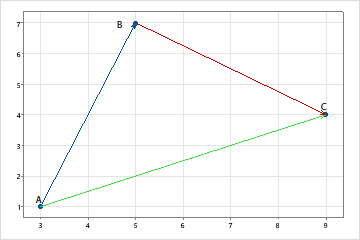# 配对斜率概述

X Y斜率 AB = (7 – 1) / (5 – 3) = 3 斜率 AC = (4 – 1) / (9 – 3) = 1/2 斜率 BC = (4 – 7) / (9 – 5) = −3/4